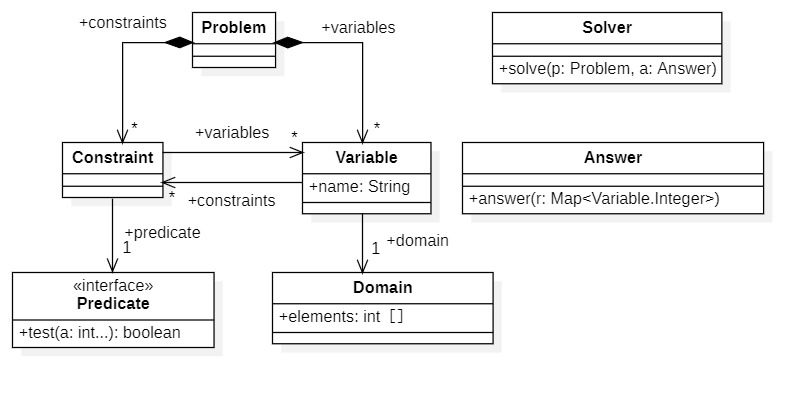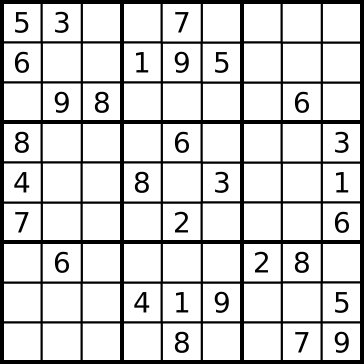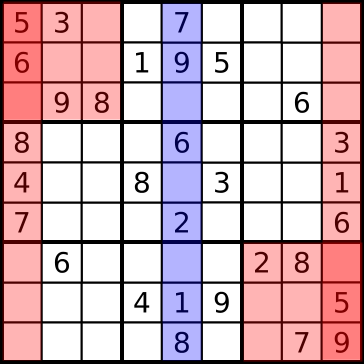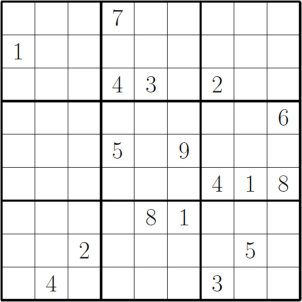# Introduction

Constraint Programming (https://en.wikipedia.org/wiki/%E5%88%B6%E7%B4%84%E3%83%97%E3%83%AD%E3%82% B0% E3% 83% A9% E3% 83% 9F% E3% 83% B3% E3% 82% B0) is one of the programming paradigms. In constraint programming, a program is written by describing the relationships between variables in the form of constraints. Nowadays, AI is said to be synonymous with deep learning, but in the past, this constraint programming and computer algebra were also called AI. Implement a constraint programming library in Java and try to solve problems such as eight queens, verbal arithmetic, and sudoku.

# Target problem

Target the simplest problems. Specifically, the problems are as follows.

• The domain of variables is only integers.
• It is assumed that the domain of a variable is finite and its domain is defined in the problem.

# Simple example

Consider a simple example for illustration.

• Find the values of variables a, b, c that satisfy the expression a + b = c.
• However, a <b.
• The domain of variables a, b, and c is {1, 2, 3}.

# approach

Since we only need to find a combination that satisfies the constraint for each value in the domain of each variable, consider a simple triple-loop program. Expressed in Java, it looks like this.

``````for (int a : List.of(1, 2, 3))
for (int b : List.of(1, 2, 3))
for (int c : List.of(1, 2, 3))
if (a < b)
if (a + b == c)
``````

ʻAnswer` is a callback function that is called when a solution is found. In this program, the innermost part of the nested for loop is executed 3 x 3 x 3 = 27 times. Considering the processing efficiency, this can be improved a little. The constraint ʻa <b` can be checked when the values of the variables ʻa`and`b` are fixed, so it can be rewritten as follows.

``````for (int a : List.of(1, 2, 3))
for (int b : List.of(1, 2, 3))
if (a < b)
for (int c : List.of(1, 2, 3))
if (a + b == c)
``````

There are only three combinations of `(a, b)` that satisfy ʻa <b`: `(1, 2), (1, 3), (2, 3)`. Since `for (int c: List.of (1, 2, 3))` is executed for each of these three ways, the innermost part of the nested for loop should be 3 x 3 = 9 times. Will be. You can expect about 3 times the processing performance compared to the above code. It may be faster as it also reduces the number of constraint checks. Let's configure the program with this approach. In summary, it looks like this.

• Nest a loop that sequentially assigns domain values to each variable.
• Constraints are checked in the outer loop as much as possible to improve efficiency.
• Call the callback function when a solution is found.

# model

The following are possible class candidates.

• Problem
A class of problems to solve.
• Variable
The variable that appears in the problem.
• Domain
The domain of each variable.
• Constraint
The constraint expression that the variable must satisfy.
• Predicate
A functional interface for expressing constraint expressions as lambda expressions.
• Problem Solving (Solver)
Solve the problem.
A callback that will be called when a solution is found.

It looks like this when expressed as a UML class diagram.# code

Domain is a list of immutable integers.

#### `Domain.java`

``````
public class Domain extends AbstractList<Integer> {

private final int[] elements;

private Domain(int... elements) {
this.elements = elements;
}

public static Domain of(int... elements) {
return new Domain(Arrays.copyOf(elements, elements.length));
}

public static Domain range(int startInclusive, int endExclusive) {
return new Domain(IntStream.range(startInclusive, endExclusive).toArray());
}

public static Domain rangeClosed(int startInclusive, int endInclusive) {
return range(startInclusive, endInclusive + 1);
}

@Override public Integer get(int index) { return elements[index]; }
@Override public int size() { return elements.length; }
}
``````

A variable is a class that has a name and a domain. It holds a reference to all the constraints on that variable. Since the instance is created by the factory method of the problem (Problem), the constructor is package scope.

#### `Variable.java`

``````
public class Variable {

public final String name;
public final Domain domain;

private final List<Constraint> _constraints = new ArrayList<Constraint>();
public final List<Constraint> constraints = Collections.unmodifiableList(_constraints);

Variable(String name, Domain domain) {
this.name = name;
this.domain = domain;
}

}

@Override
public String toString() {
return name;
}

}
``````

A constraint expression (Predicate) is a function interface for expressing a constraint in an expression. Only the `test (int ...)` method, which is called with the value of each variable as an argument when the values of all variables related to the constraint are bound, is defined. You can use this to express a constraint expression as a lambda expression.

#### `Predicate.java`

``````
@FunctionalInterface
public interface Predicate {

boolean test(int... values);

}
``````

A constraint (Predicate) is an immutable class that has a constraint expression (Predicate) and a reference to the variable related to the constraint. Since the instance is created by the factory method of the problem (Problem), the constructor is package scope.

#### `Constraint.java`

``````
public class Constraint {

public final Predicate predicate;
public final List<Variable> variables;

Constraint(Predicate predicate, Variable... variables) {
this.predicate = predicate;
this.variables = List.of(variables);
}

@Override
public String toString() {
return "Constraint" + variables;
}
}
``````

A problem is a class with all relevant variables and constraints. Variables are defined by `variable (String, Domain)`. Constraints are defined with `constraint (Predicate, Variable ...)`. For multiple variables, there is a method ʻallDifferent (Variable ...)` to easily express the constraint that each two variables have different values.

#### `Problem.java`

``````
public class Problem {

private List<Variable> _variables = new ArrayList<>();
public List<Variable> variables = Collections.unmodifiableList(_variables);
private Map<String, Variable> variableMap = new HashMap<>();

private List<Constraint> _constraints = new ArrayList<>();
public List<Constraint> constraints = Collections.unmodifiableList(_constraints);

public Variable variable(String name, Domain domain) {
if (variableMap.containsKey(name))
throw new IllegalArgumentException("Duplicate variable name: " + name);
Variable v = new Variable(name, domain);
this.variableMap.put(name, v);
return v;
}

public Variable variable(String name) {
return variableMap.get(name);
}

public Constraint constraint(Predicate predicate, Variable... variables) {
Constraint c = new Constraint(predicate, variables);
for (Variable v : variables)
return c;
}

public void allDifferent(Variable... variables) {
for (int i = 0, size = variables.length; i < size; ++i)
for (int j = i + 1; j < size; ++j)
constraint(a -> a != a, variables[i], variables[j]);
}

}
``````

Answer is a callback function to receive the found solution. Receives a variable-value pair as a Map. Since it is essentially a function interface, you can write callback functions in lambda expressions.

#### `Answer.java`

``````

}

``````

Solver has a `solve (Problem, Answer)` method that finds a solution from a problem. Since the number of variables is variable, it is not possible to realize binding by nesting for statements as described at the beginning, so binding is performed by a recurrence call. The overloaded `solve (Problem, List <Variable>, Answer)` method allows you to specify the order in which variables are bound with `List <Variable>` when solving a problem. The internal static method `constraintOrder (List <Variable>, List <Constraint>)` finds the order of applicable constraints (Constraint) from the bound order of variables.

#### `Solver.java`

``````
public class Solver {

static final Logger logger = Logger.getLogger(Solver.class.getName());

static List<List<Constraint>> constraintOrder(List<Variable> bindingOrder, List<Constraint> constraints) {
int variableSize = bindingOrder.size();
int constraintSize = constraints.size();
List<List<Constraint>> result = new ArrayList<>(variableSize);
Set<Constraint> done = new HashSet<>(constraintSize);
Set<Variable> bound = new HashSet<>(variableSize);
for (Variable v : bindingOrder) {
List<Constraint> list = new ArrayList<>();
for (Constraint c : constraints)
if (!done.contains(c) && bound.containsAll(c.variables)) {
}
}
return result;
}

int variableSize = problem.variables.size();
List<List<Constraint>> constraintOrder = constraintOrder(bindingOrder, problem.constraints);
int[] arguments = new int[variableSize];
Map<Variable, Integer> result = new LinkedHashMap<>(variableSize);

new Object() {

boolean test(int i) {
for (Constraint c : constraintOrder.get(i)) {
int p = 0;
for (Variable v : c.variables)
arguments[p++] = result.get(v);
if (!c.predicate.test(arguments))
return false;
}
return true;
}

void solve(int i) {
if (i >= variableSize)
else {
Variable v = bindingOrder.get(i);
Domain d = v.domain;
for (int value : d) {
result.put(v, value);
if (test(i))
solve(i + 1);
}
}
}

}.solve(0);
}

}

}
``````

# test

Let's actually solve the simple example at the beginning.

``````Problem problem = new Problem();
Domain domain = Domain.of(1, 2, 3);
Variable A = problem.variable("A", domain);
Variable B = problem.variable("B", domain);
Variable C = problem.variable("C", domain);
Constraint X = problem.constraint(a -> a + a == a, A, B, C);
Constraint Y = problem.constraint(a -> a < a, A, B);
Solver solver = new Solver();
solver.solve(problem, result -> System.out.println(result));
``````

The result is like this.

``````{A=1, B=2, C=3}
``````

The bound order of variables and the application order of constraints are as follows.

0: A [] 1: B [Constraint [A, B]] 2: C [Constraints [A, B, C]]

# Eight queens

[Eight Queens-Wikipedia](https://ja.wikipedia.org/wiki/%E3%82%A8%E3%82%A4%E3%83%88%E3%83%BB%E3%82%AF % E3% 82% A4% E3% 83% BC% E3% 83% B3) is a problem of placing 8 queens on the chess board. At this time, none of the pieces should be in a position where they can be taken by other pieces. The queen moves in eight directions, up, down, left, right, diagonally, as long as there are no obstacles. It is a movement that combines the rook of shogi and the bishop. A modified version with n on one side is called an "n-queen" puzzle. Prepare n variables whose domain is \$ \ {1..n \} \$, and solve them as a problem so that they are different from each other and do not overlap diagonally. Here, we will find the number of solutions for \$ n ∊ \ {1, 2, 3, 4, 5, 6, 7, 8, 9, 10 \} \$.

``````class TestNQueens {

static Logger logger = Logger.getLogger(TestNQueens.class.getName());

static int nQueens(final int n) {
long start = System.currentTimeMillis();
Problem problem = new Problem();
Domain domain = Domain.range(0, n);
Variable[] rows = IntStream.range(0, n)
.mapToObj(i -> problem.variable("R" + i, domain))
.toArray(Variable[]::new);
for (int i = 0; i < n; ++i)
for (int j = i + 1; j < n; ++j) {
int distance = j - i;
problem.constraint(
(x, y) -> x != y && Math.abs(x - y) != distance, rows[i], rows[j]);
}
Solver solver = new Solver();
+ " : elapse=" + (System.currentTimeMillis() - start) + "ms.");
}

@Test
void test() {
assertEquals(1, nQueens(1));
assertEquals(0, nQueens(2));
assertEquals(0, nQueens(3));
assertEquals(2, nQueens(4));
assertEquals(10, nQueens(5));
assertEquals(4, nQueens(6));
assertEquals(40, nQueens(7));
assertEquals(92, nQueens(8));
assertEquals(352, nQueens(9));
assertEquals(724, nQueens(10));
}

}
``````

The result looks like this. It matched the description on Wikipedia.

``````2020-05-19T16:31:06.863 Information n=1 : answers=1 : elapse=27ms.
2020-05-19T16:31:06.941 Information n=2 : answers=0 : elapse=3ms.
2020-05-19T16:31:06.942 Information n=3 : answers=0 : elapse=0ms.
2020-05-19T16:31:06.944 Information n=4 : answers=2 : elapse=0ms.
2020-05-19T16:31:06.947 Information n=5 : answers=10 : elapse=2ms.
2020-05-19T16:31:06.953 Information n=6 : answers=4 : elapse=5ms.
2020-05-19T16:31:06.963 Information n=7 : answers=40 : elapse=10ms.
2020-05-19T16:31:06.984 Information n=8 : answers=92 : elapse=20ms.
2020-05-19T16:31:07.031 Information n=9 : answers=352 : elapse=45ms.
2020-05-19T16:31:07.118 Information n=10 : answers=724 : elapse=87ms.
``````

# Verbal arithmetic

Next, let's solve the verbal arithmetic. It is the famous SEND MORE MONEY. Assign a one-digit number to each letter so that the formula holds. The same alphabet is the same number, different alphabets are different numbers, and the first digit is non-zero.

``````  SEND
+ MORE
------
MONEY
``````
``````    static Logger logger = Logger.getLogger(TestSendMoreMoney.class.getName());

static int number(int... digits) {
return IntStream.of(digits).reduce(0, (t, d) -> t * 10 + d);
}

@Test
public void test single constraint() {
Problem problem = new Problem();
Domain first = Domain.of(1, 2, 3, 4, 5, 6, 7, 8, 9);
Domain rest = Domain.of(0, 1, 2, 3, 4, 5, 6, 7, 8, 9);
Variable S = problem.variable("S", first);
Variable E = problem.variable("E", rest);
Variable N = problem.variable("N", rest);
Variable D = problem.variable("D", rest);
Variable M = problem.variable("M", first);
Variable O = problem.variable("O", rest);
Variable R = problem.variable("R", rest);
Variable Y = problem.variable("Y", rest);
Variable[] variables = {S, E, N, D, M, O, R, Y};
problem.allDifferent(variables);
problem.constraint(a ->
number(a, a, a, a) + number(a, a, a, a)
== number(a, a, a, a, a), variables);
Solver solver = new Solver();
solver.solve(problem, m -> logger.info(m.toString()));
}
``````

The result is like this.

``````{S=9, E=5, N=6, D=7, M=1, O=0, R=8, Y=2}
``````

This solution has only one constraint and is checked after all variables are bound. In other words, it is not very efficient because the constraint check is done in the innermost loop. It took less than 2 seconds in my environment. It's a little faster if you add a carry variable and define a constraint for each digit.

``````    @Test
public void test Digit-by-digit constraint() {
Domain zero = Domain.of(0);
Domain first = Domain.of(1, 2, 3, 4, 5, 6, 7, 8, 9);
Domain rest = Domain.of(0, 1, 2, 3, 4, 5, 6, 7, 8, 9);
Domain carry = Domain.of(0, 1);
Problem problem = new Problem();
Variable Z = problem.variable("Z", zero);
Variable C1 = problem.variable("C1", carry);
Variable C2 = problem.variable("C2", carry);
Variable C3 = problem.variable("C3", carry);
Variable C4 = problem.variable("C4", carry);
Variable S = problem.variable("S", first);
Variable E = problem.variable("E", rest);
Variable N = problem.variable("N", rest);
Variable D = problem.variable("D", rest);
Variable M = problem.variable("M", first);
Variable O = problem.variable("O", rest);
Variable R = problem.variable("R", rest);
Variable Y = problem.variable("Y", rest);
Variable[] variables = {S, E, N, D, M, O, R, Y};
problem.allDifferent(variables);
//  C4 C3 C2 C1  Z
//   Z  S  E  N  D
// + Z  M  O  R  E
// ---------------
//   M  O  N  E  Y
Predicate digitPredicate = a -> a + a + a == a + a * 10;
problem.constraint(digitPredicate, Z, D, E, Y, C1);
problem.constraint(digitPredicate, C1, N, R, E, C2);
problem.constraint(digitPredicate, C2, E, O, N, C3);
problem.constraint(digitPredicate, C3, S, M, O, C4);
problem.constraint(digitPredicate, C4, Z, Z, M, Z);
Solver solver = new Solver();
solver.solve(problem, m -> logger.info(m.toString()));
}
``````

It can be solved in about 0.3 seconds.

# Sudoku

Let's solve the first problem in Sudoku-Wikipedia.``````    static Logger logger = Logger.getLogger(Test Sudoku.class.toString());

static int Edge length= 9;
static int Side length of small rectangle= 3;
static Domain domain= Domain.rangeClosed(1, 9);

static String name(int r, int c) {
return r + "@" + c;
}

static Variable[][]Variable definition(Problem problem, int[][]input) {
Variable[][]variable= new Variable[Side length][Side length];
for (int r = 0; r <Side length; ++r)
for (int c = 0; c <Side length; ++c)
variable[r][c] =problem.variable(name(r, c),
input[r][c] == 0 ?Domain: Domain.of(input[r][c]));
return variable;
}

static List<Variable[]>Constraint definition(Problem problem, Variable[][]variable) {
List<Variable[]>Constraint variable= new ArrayList<>();
for (int r = 0; r <Side length; ++r)
for (int c = 0; c <Side length; ++c) {
Variable[] va = new Variable[Side length];
for (int r = 0; r <Side length; ++r)
va[r] =variable[r][c];
}
for (int r = 0; r <Side length; r +=Side length of small rectangle)
for (int c = 0; c <Side length; c +=Side length of small rectangle) {
Variable[] va = new Variable[Side length];
for (int i = 0, p = 0; i <Side length of small rectangle; ++i)
for (int j = 0; j <Side length of small rectangle; ++j, ++p)
va[p] =variable[r + i][c + j];
}
for (Variable[] va :Constraint variable)
problem.allDifferent(va);
return constraint variable;
}

for (int r = 0; r <Side length; ++r) {
StringBuilder sb = new StringBuilder();
for (int c = 0; c <Side length; ++c)
logger.info(sb.toString());
}
}

static void Sudoku binding order not specified(int[][]input) {
Problem problem= new Problem();
Variable[][]variable=Variable definition(problem,input);
Constraint definition(problem,variable);
Solver solver= new Solver();
}

@Test
void testWikipedia No binding order specified() {
//Wikipedia Sudoku example
// https://ja.wikipedia.org/wiki/%E6%95%B0%E7%8B%AC
int[][]input= {
{ 5, 3, 0, 0, 7, 0, 0, 0, 0 },
{ 6, 0, 0, 1, 9, 5, 0, 0, 0 },
{ 0, 9, 8, 0, 0, 0, 0, 6, 0 },
{ 8, 0, 0, 0, 6, 0, 0, 0, 3 },
{ 4, 0, 0, 8, 0, 3, 0, 0, 1 },
{ 7, 0, 0, 0, 2, 0, 0, 0, 6 },
{ 0, 6, 0, 0, 0, 0, 2, 8, 0 },
{ 0, 0, 0, 4, 1, 9, 0, 0, 5 },
{ 0, 0, 0, 0, 8, 0, 0, 7, 9 },
};
logger.info("test wikipedia");
Sudoku binding order not specified(input);
}

``````

I solved it for the time being, but it took more than 20 seconds.

``````2020-05-16T21:01:31.789 info test wikipedia
2020-05-16T21:01:52.360 Information 5 3 4 6 7 8 9 1 2
2020-05-16T21:01:52.361 Information 6 7 2 1 9 5 3 4 8
2020-05-16T21:01:52.361 Information 1 9 8 3 4 2 5 6 7
2020-05-16T21:01:52.362 Information 8 5 9 7 6 1 4 2 3
2020-05-16T21:01:52.363 Information 4 2 6 8 5 3 7 9 1
2020-05-16T21:01:52.363 Information 7 1 3 9 2 4 8 5 6
2020-05-16T21:01:52.363 Information 9 6 1 5 3 7 2 8 4
2020-05-16T21:01:52.364 Information 2 8 7 4 1 9 6 3 5
2020-05-16T21:01:52.365 Information 3 4 5 2 8 6 1 7 9
``````

The bound order of variables is simply top to bottom and left to right, so let's devise a little. The binding order is defined by the following policy.

1. Bind the squares with the determined numbers first. These squares have no backtracking with fixed values.
2. Look at each row, column, and 3x3 area, and bind in order from the place with the most numbered squares. As shown in the figure below, the area shown in blue has 6 fixed values. Five values are fixed for the four areas shown in red. Bind variables in the blue area → variables in the red area → ...The following code changes the bound order of variables according to this policy.

``````    static List<Variable>Binding order definition(List<Variable>variable, List<Variable[]>Constraint variable) {
for (Variable v :variable)
if (v.domain.size() == 1)
Collections.sort(Constraint variable,
Comparator.comparingInt(a -> Arrays.stream(a).mapToInt(v -> v.domain.size()).sum()));
for (Variable[] a :Constraint variable)
for (Variable v : a)
return new ArrayList<>(Binding order);
}

static void Sudoku binding order specified(int[][]input) {
Problem problem= new Problem();
Variable[][]variable=Variable definition(problem,input);
List<Variable[]>Constraint variable=Constraint definition(problem,variable);
List<Variable>Binding order=Binding order definition(problem.variables,Constraint variable);
Solver solver= new Solver();
}
``````

As a result, it can be solved in about 0.02 seconds. This example is too simple, so I tried a more difficult problem. According to Wikipedia, 17 or more squares with fixed numbers are required for Sudoku's solution to be unique. I searched on the net and picked up exactly 17 difficult problems with fixed numbers.``````    @Test
void testGood_at_Sudoku_Heres_some_youll_never_complete() {
// http://theconversation.com/good-at-sudoku-heres-some-youll-never-complete-5234
int[][]input= {
{ 0, 0, 0, 7, 0, 0, 0, 0, 0 },
{ 1, 0, 0, 0, 0, 0, 0, 0, 0 },
{ 0, 0, 0, 4, 3, 0, 2, 0, 0 },
{ 0, 0, 0, 0, 0, 0, 0, 0, 6 },
{ 0, 0, 0, 5, 0, 9, 0, 0, 0 },
{ 0, 0, 0, 0, 0, 0, 4, 1, 8 },
{ 0, 0, 0, 0, 8, 1, 0, 0, 0 },
{ 0, 0, 2, 0, 0, 0, 0, 5, 0 },
{ 0, 4, 0, 0, 0, 0, 3, 0, 0 },
};
logger.info("Good at Sudoku Heres some youll never complete");
Sudoku binding order specified(input);
}
``````

I was able to solve it within 1 second.

``````2020-05-16T21:22:26.176 Information Good at Sudoku Heres some youll never complete
2020-05-16T21:22:26.310 Information 2 6 4 7 1 5 8 3 9
2020-05-16T21:22:26.311 Information 1 3 7 8 9 2 6 4 5
2020-05-16T21:22:26.312 Information 5 9 8 4 3 6 2 7 1
2020-05-16T21:22:26.313 Information 4 2 3 1 7 8 5 9 6
2020-05-16T21:22:26.315 Information 8 1 6 5 4 9 7 2 3
2020-05-16T21:22:26.316 Information 7 5 9 6 2 3 4 1 8
2020-05-16T21:22:26.317 Information 3 7 5 2 8 1 9 6 4
2020-05-16T21:22:26.318 Information 9 8 2 3 6 4 1 5 7
2020-05-16T21:22:26.320 Information 6 4 1 9 5 7 3 8 2
``````

If you did not specify the bound order of the variables, it could not be solved even after 10 minutes.

# Improvement of constraint expression

When you define a constraint, you specify variables that are associated with your lambda expression. This is how it is written because the number of variables subject to constraints is variable.

``````        problem.constraint(a ->
number(a, a, a, a)
+ number(a, a, a, a)
== number(a, a, a, a, a),
S, E, N, D, M, O, R, Y);
``````

There is a correspondence between ʻa `to`S`, ʻa ` to ʻE`, and so on, but the expression is difficult to understand. To improve this, add a fixed-length argument method. First of all, add the following interface.

``````@FunctionalInterface
public interface Predicate1 extends Predicate {

default boolean test(int... values) {
return test(values);
}

boolean test(int a);

}

@FunctionalInterface
public interface Predicate2 extends Predicate {

default boolean test(int... values) {
return test(values, values);
}

boolean test(int a, int b);

}

.....
``````

Then add a factory method for the constraint that uses it to the Problem class.

#### `Problem.java`

``````
public Constraint constraint(Predicate1 predicate, Variable a) {
return constraint((Predicate)predicate, a);
}

public Constraint constraint(Predicate2 predicate, Variable a, Variable b) {
return constraint((Predicate)predicate, a, b);
}

.....
``````

Then you will be able to write like this. If the number of `Variable`s passed to the`constraint ()`method and the number of arguments of the lambda expression do not match, a compile error will occur.

``````        problem.constraint((s, e, n, d, m, o, r, y) ->
number(s, e, n, d)
+ number(m, o, r, e)
== number(m, o, n, e, y),
S, E, N, D, M, O, R, Y);
``````

# Summary

We found that the following points affect the performance.

1. ** How to give constraints **
Finer constraints so that you can check variable bindings early will speed up your choices.
2. ** Order of variable binding **
It will be faster if you bind variables with few choices first.